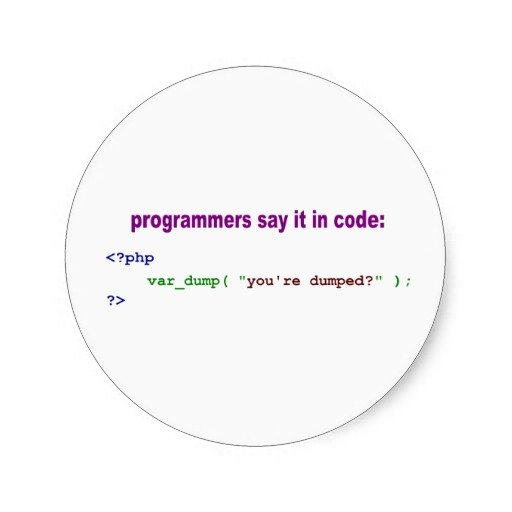# Round and Format number in PHP

Making Round and Format number in PHP is quite easy but it will take you much time if you are not familiar with it. But here we help you solve all of these type of issues just in 5 minutes :).[code]float round ( float \$val [, int \$precision = 0 [, int \$mode = PHP_ROUND_HALF_UP ]] )[/code] [code]string number_format ( float \$number , int \$decimals = 0 , string \$dec_point = “.” , string \$thousands_sep = “,” )[/code]

Now, let ‘s start

## 1. Round number in PHP

It ‘s easier than you thought, we have some basic ways to use it here

Keep in mind that, the difference from round and format is the value. When you try to format a number, it only mean you show it to user the different way, but when you use round, you already change the value to value you want. We will talk more about format later, here if you want to get 3 numbers after decimal point, you can use like this

It will give you 2.412

I think that ‘s all you need to round a number in PHP

## 2. Format number in PHP

Let ‘s continue with format number in PHP by an simple example

And I believe you know how to use after looking at this example.